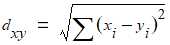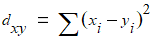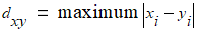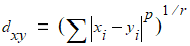# NMath User's Guide

46.3 Hierarchical Cluster Analysis (.NET, C#, CSharp, VB, Visual Basic, F#)

Cluster analysis detects natural groupings in data. In hierarchical cluster analysis, each object is initially assigned to its own singleton cluster. The analysis then proceeds iteratively, at each stage joining the two most similar clusters into a new cluster, continuing until there is one overall cluster. In NMath Stats, class performs hierarchical cluster analyses.

Distance Functions

During clustering, the distance between individual objects is computed using a distance function. The distance function is encapsulated in a Distance.Function delegate, which takes two vectors and returns a measure of the distance (similarity) between them:

Code Example – C# hierarchical cluster analysis

```public delegate double Function( DoubleVector data1,
```
```                                 DoubleVector data2 );
```

Code Example – VB hierarchical cluster analysis

```Delegate Function(Data1 As DoubleVector, Data2 As DoubleVector) As
```
```  Double
```

Delegates are provided as static variables on class Distance for many common distance functions:

Distance.EuclideanFunction computes the Euclidean distance between two data vectors (2 norm):Euclidean distance is simply the geometric distance in the multidimen­sional space.

Distance.SquaredEuclideanFunction computes the squared Euclidean distance between two vectors:Squaring the simple Euclidean distance places progressively greater weight on objects that are further apart.

Distance.CityBlockFunction computes the city-block (Manhattan) distance between two vectors (1 norm):In most cases, the city-block distance measure yields results similar to the simple Euclidean distance. Note, however, that the effect of outliers is dampened, since they are not squared.

Distance.MaximumFunction computes the maximum (Chebychev) distance between two vectors:This distance measure may be appropriate in cases when you want to define two objects as different if they differ on any one of the dimensions.

Distance.PowerFunction( double p, double r ) computes the power distance between two vectors:where p and r are user-defined parameters. Parameter p controls the pro­gressive weight that is placed on differences on individual dimensions; parameter r controls the progressive weight that is placed on larger differ­ences between objects. Appropriate selections of p and r yield Euclidean, squared Euclidean, Minkowski, city-block, and many other distance met­rics. For example, if p and r are equal to 2, the power distance is equal to the Euclidean distance.

All provided distance functions allow missing values. Pairs of elements are excluded from the distance measure when their comparison returns NaN. If all pairs are excluded, NaN is returned for the distance measure.

You can also define your own Distance.Function delegate and use it to cluster your data. For example, if you have function MyDistance() that computes the distance between two vectors:

Code Example – C# hierarchical cluster analysis

```public double MyDistance( DoubleVector x, DoubleVector y );
```

Code Example – VB hierarchical cluster analysis

```Public Function MyDistance(X As DoubleVector, Y As DoubleVector) As
```
```  Double
```

You can define a Distance.Function delegate like so:

Code Example – C# hierarchical cluster analysis

```var MyDistanceFunction = new Distance.Function( MyDistance );
```

Code Example – VB hierarchical cluster analysis

```Dim MyDistanceFunction As New Distance.Function(AddressOf
```
```  MyDistance)
```

During clustering, the distances between clusters of objects are computed using a linkage function. The linkage function is encapsulated in a Linkage.Function delegate. When two groups P and Q are united, a linkage function computes the distance between the new combined group P + Q and another group R.

Figure 6 – Computing the distance between clusters using a linkage functionThe parameters to the Linkage.Function—which may not necessarily all be used to calculate the result—are the distance between R and P, the distance between R and Q, the distance between P and Q, and the sizes (n) of all three groups:

Code Example – C# hierarchical cluster analysis

```public delegate double Function( double Drp, double Drq,
```
```  double Dpq, double Nr, double Np, double Nq );
```

Code Example – VB hierarchical cluster analysis

```Delegate Function(DRP As Double, DRQ As Double,
```
```  DPQ As Double, NR As Double, NP As Double, NQ As Double) As
```
```  Double
```

Delegates are provided as static variables on class Linkage for many common linkage functions:

Linkage.SingleFunction computes the distance between two clusters as the distance of the two closest objects (nearest neighbors) in the clusters. Adopting a friends-of-friends clustering strategy closely related to the minimal spanning tree, the single linkage method tends to result in long chains of clusters.

Linkage.CompleteFunction computes the distance between two clusters as the greatest distance between any two objects in the different clusters (furthest neighbors). The complete linkage method tends to work well in cases where objects form naturally distinct clumps.

Linkage.UnweightedAverageFunction computes the distance between two clusters as the average distance between all pairs of objects in the two different clusters. This method is sometimes referred to as unweighted pair-group method using arithmetic averages, and abbreviated UPGMA.

Linkage.WeightedAverageFunction computes the distance between two clusters as the average distance between all pairs of objects in the two different clusters, using the size of each cluster as a weighting factor. This method is sometimes referred to as weighted pair-group method using arithmetic averages, and abbreviated WPGMA.

Linkage.CentroidFunction computes the distance between two clusters as the difference between centroids. The centroid of a cluster is the average point in the multidimensional space. The centroid method is sometimes referred to as unweighted pair-group method using the centroid average, and abbreviated UPGMC.

Linkage.MedianFunction computes the distance between two clusters as the difference between centroids, using the size of each cluster as a weighting factor. This is sometimes referred to as weighted pair-group method using the centroid average, and abbreviated WPGMC.

Linkage.WardFunction computes the distance between two clusters using Ward's method. Ward's method uses an analysis of variance approach to evaluate the distances between clusters. The smaller the increase in the total within-group sum of squares as a result of joining two clusters, the closer they are. The within-group sum of squares of a cluster is defined as the sum of the squares of the distance between all objects in the cluster and the centroid of the cluster. Ward's method tends to produce compact groups of well-distributed size.

You can also define your own Linkage.Function delegate and use it to cluster your data. For example, if you have function MyLinkage() that computes the distance between two clusters:

Code Example – C# hierarchical cluster analysis

```public double MyLinkage( double Drp, double Drq, double Dpq,
```
```                         double Nr, double Np, double Nq );
```

Code Example – VB hierarchical cluster analysis

```Public Function MyLinkage(DRP As Double, DRQ As Double, DPQ As
```
```  Double, NR As Double, NP As Double, NQ As Double) As Double
```

You can define a Linkage.Function delegate like so:

Code Example – C# hierarchical cluster analysis

```var MyLinkageFunction = new Linkage.Function( MyLinkage );
```

Code Example – VB hierarchical cluster analysis

```Dim MyLinkageFunction As New Linkage.Function(AddressOf MyLinkage)
```

Creating Cluster Analyses

A instance is constructed from a matrix or a dataframe containing numeric data. Each row in the data set represents an object to be clustered.

Code Example – C# hierarchical cluster analysis

```var ca = new ClusterAnalysis( data );
```

Code Example – VB hierarchical cluster analysis

```Dim CA As New ClusterAnalysis(Data)
```

The current default distance and linkage delegates are used. The default distance and linkage delegates are Distance.EuclideanFunction and Linkage.SingleFunction, unless the defaults have been changed using the static DefaultDistanceFunction and DefaultLinkageFunction properties. For example:

Code Example – C# hierarchical cluster analysis

```ClusterAnalysis.DefaultDistanceFunction = Distance.MaximumFunction;
```
```ClusterAnalysis.DefaultLinkageFunction = Linkage.CentroidFunction;
```

Code Example – VB hierarchical cluster analysis

```ClusterAnalysis.DefaultDistanceFunction = Distance.MaximumFunction
```
```ClusterAnalysis.DefaultLinkageFunction = Linkage.CentroidFunction
```

This changes the default distance and linkage functions for all subsequently constructed ClusterAnalysis objects.

You can also specify non-default distance and linkage functions in the constructor:

Code Example – C# hierarchical cluster analysis

```var ca = new ClusterAnalysis( data,
```
```  Distance.PowerFunction( 1.25, 2.0 ), Linkage.CompleteFunction );
```

Code Example – VB hierarchical cluster analysis

```Dim CA As New ClusterAnalysis(Data,
```
```  Distance.PowerFunction(1.25, 2.0), Linkage.CompleteFunction)
```

After construction, you can retrieve information about the ClusterAnalysis configuration using the provided properties:

N gets the total number of objects being clustered.

DistanceFunction gets and sets the distance function delegate used to measure the distance between individual objects. Setting the distance function using the DistanceFunction property has no effect until Update() is called with new data. (See below.)

LinkageFunction gets and sets the linkage function used to measure the distance between clusters of objects. Setting the linkage delegate using the LinkageFunction property has no effect until Update() is called with new data. (See below.)

Cluster Analysis Results

The Distances property gets the vector of distances between all possible object pairs, computed using the current distance delegate. For n objects, the distance vector is of length (n-1)(n/2), with distances arranged in the order:

```(1,2), (1,3), ..., (1,n), (2,3), ..., (2,n), ..., ..., (n-1,n)
```

Linkages gets an (n-1) x 3 matrix containing the complete hierarchical linkage tree, computed from Distances using the current linkage delegate. At each level in the tree, columns 1 and 2 contain the indices of the clusters linked to form the next cluster. Column 3 contains the distances between the clusters. For example, this code clusters 8 random vectors of length 3, then shows a sample output of the hierarchical cluster tree:

Code Example – C# hierarchical cluster analysis

```var data = new DoubleMatrix( 8, 3, new RandGenUniform() );
```
```var ca = new ClusterAnalysis( data );
```
```Console.WriteLine( ca.Linkages );
```

Code Example – VB hierarchical cluster analysis

```Dim Data As New DoubleMatrix(8, 3, New RandGenUniform())
```
```Dim CA As New ClusterAnalysis(Data)
```
```Console.WriteLine(ca.Linkages)
```

Sample output:

```7x3 [
```
```      4 7 0.194409151975696
```
```      3 5 0.290431894003636
```
```      2 9 0.495557235783239
```
```      1 6 0.508966210536187
```
```      0 11 0.522321103698264
```
```      8 10 0.590187697768796
```
```      12 13 0.621675638177606 ]
```

Each object is initially assigned to its own singleton cluster, numbered 0 to 7. The analysis then proceeds iteratively, at each stage joining the two most similar clusters into a new cluster, continuing until there is one overall cluster. The first new cluster formed by the linkage function is assigned index 8, the second is assigned index 9, and so forth. When these indices appear later in the tree, the clusters are being combined again into a still larger cluster.

The CutTree() method constructs a set of clusters by cutting the hierarchical linkage tree either at the specified height, or into the specified number of clusters. For example, this code cuts the linkage tree to form 3 clusters:

Code Example – C# hierarchical cluster analysis

```ca.CutTree( 3 );
```

Code Example – VB hierarchical cluster analysis

```CA.CutTree(3)
```

This code cuts the linkage tree at a height of 0.75:

Code Example – C# hierarchical cluster analysis

```ca.CutTree( 0.75 );
```

Code Example – VB hierarchical cluster analysis

```CA.CutTree(0.75)
```

The CutTree() method returns a object, which represents a collection of objects assigned to a finite number of clusters. The NumberOfClusters property

gets the number of clusters into which objects are grouped; N gets the number of objects. The Clusters property returns an array of integers that identifies the cluster into which each object was grouped. Cluster numbers are arbitrary, and range from 0 to NumberOfClusters - 1. The indexer gets the cluster to which a given object is assigned. The Cluster() method returns the objects assigned to a given cluster as an array of integers. For instance:

Code Example – C# hierarchical cluster analysis

```// Cluster 10 random vectors of length 4:
```
```var data = new DoubleMatrix( 10, 4, new RandGenUniform() );
```
```var ca = new ClusterAnalysis( data );

```

```// Cut the tree into 5 clusters
```
```ClusterSet cut = ca.CutTree( 5 );

```

```Console.WriteLine( "ClusterSet = " + cut );
```
```Console.WriteLine( "Object 0 is in cluster: " + cut );
```
```Console.WriteLine( "Object 3 is in cluster: " + cut );
```
```Console.WriteLine( "Object 8 is in cluster: " + cut );
```
```int[] objects = cut.Cluster( 1 );
```
```Console.Write( "Objects in cluster 1: " );
```
```for (int i = 0; i < objects.Length; i++ )
```
```{
```
```  Console.Write( objects[i] + " " );
```
```}
```
```Console.WriteLine();
```

Code Example – VB hierarchical cluster analysis

```'' Cluster 10 random vectors of length 4:
```
```Dim Data As New DoubleMatrix(10, 4, New RandGenUniform())
```
```Dim ca As New ClusterAnalysis(Data)

```

```'' Cut the tree into 5 clusters
```
```Dim Cut As ClusterSet = CA.CutTree(5)

```

```Console.WriteLine("ClusterSet = " & cut)
```
```Console.WriteLine("Object 0 is in cluster: " & Cut(0))
```
```Console.WriteLine("Object 3 is in cluster: " & Cut(3))
```
```Console.WriteLine("Object 8 is in cluster: " & Cut(8))
```
```Dim Objects() As Integer = Cut.Cluster(1)
```
```Console.Write("Objects in cluster 1: ")
```
```For I As Integer = 0 To Objects.Length - 1
```
```  Console.Write(Objects(I) & " ")
```
```Next
```
```Console.WriteLine()
```

Sample output:

```ClusterSet = 0,1,2,1,1,1,3,1,4,1
```
```Object 0 is in cluster: 0
```
```Object 3 is in cluster: 1
```
```Object 8 is in cluster: 4
```
```Objects in cluster 1: 1 3 4 5 7 9
```

Lastly, the CopheneticDistances property on class ClusterAnalysis gets the vector of cophenetic distances between all possible object pairs. The cophenetic distance between two objects is defined to be the intergroup distance when the objects are first combined into a single cluster in the linkage tree. The format is the same as the distance vector returned by Distances.

The correlation between the original Distances and the CopheneticDistances is sometimes taken as a measure of the appropriateness of a cluster analysis relative to the original data:

Code Example – C# hierarchical cluster analysis

```var ca = new ClusterAnalysis( data );
```
```double r = StatsFunctions.Correlation( ca.Distances,
```
```                                       ca.CopheneticDistances );
```

Code Example – VB hierarchical cluster analysis

```Dim CA As New ClusterAnalysis(Data)
```
```Dim R As Double = StatsFunctions.Correlation(CA.Distances,
```
``` CA.CopheneticDistances)
```

Reusing Cluster Analysis Objects

Method Update() updates an existing ClusterAnalysis instance with new data, and optionally with new distance and linkage functions. For example:

Code Example – C# hierarchical cluster analysis

```var ca = new ClusterAnalysis( data, Linkage.SingleFunction );
```
```Console.WriteLine( ca.Linkages );

```

```ca.Update( data, Linkage.CompleteFunction );
```
```Console.WriteLine( ca.Linkages );
```

Code Example – VB hierarchical cluster analysis

```Dim CA As New ClusterAnalysis(Data, Linkage.SingleFunction)
```
```Console.WriteLine(CA.Linkages)

```

```CA.Update(Data, Linkage.CompleteFunction)
```
```Console.WriteLine(CA.Linkages)
```

Top

Top